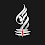Head Loss in Pipe Flow I Major and Minor losses in Pipe - VIVEK DHAKAL

# Head Loss in Pipe Flow I Major and Minor losses in Pipe

### Concept of Head Loss in Pipe Flow

When a fluid under pressure flows through a pipe, the pipe offers resistance to the flow due to which total head (or energy) of flowing fluid gets reduced by certain amount which is called head loss $(h_L)$ in pipe flow. The head loss in fluid flow may occur due to several reasons which are mainly divided into two parts as:
The head loss in fluid flow due to friction is called major head loss. It is also called friction loss $(h_f)$. The head loss in fluid flow caused by various factors such as bend in pipe, change in pipe diameter, presence of valves and fittings, etc are termed as minor head loss $(h_m)$. It is called so because its value is small in relation to friction loss for a large length of pipe. However, in case of short pipe, minor loss may become greater than the friction loss. The total head loss in the pipe is sum of major loss and minor losses.

### Major Loss / Friction Loss in Pipe Flow

The friction loss in a pipe of length $(l)$ and diameter (d) is generally calculated using Darcy-Weibach Equation as below:
$$h_f=\frac{flv^2}{2gd}$$
where,
f = friction factor
l = length of pipe
v = velocity of flow in the pipe
g = acceleration due to gravity
= $9.81 m/s^2$
d = diameter of pipe

The friction factor is evaluated depending upon the regime of flow (laminar or turbulent) and the type of surface boundary (smooth or rough). The friction factor for pipe flow is determined using Moody diagram for which Reynold's Number, pipe roughness, and diameter of pipe is required to be known. Moody diagram can be used for laminar as well as turbulent flow regime. Alternatively,  for laminar flow, friction factor can be formulated as below:
$$f=\frac{64}{Re}$$
Here, the Renyold's Number (Re) can be calculated as below:
$$Re=\frac{v\rho d}{\mu}$$
$$Re=\frac{vd}{\nu}$$
where, kinematic viscosity $(\nu)$ for water is approximately equal to $10^{-6}m^2/s$ and is formulated as below:
$$\nu=\frac{\mu}{\rho}$$
In case of turbulent flow, friction factor is determined based on whether the pipe is hydrodynamically smooth or rough. For turbulent flow in hydrodynamically smooth pipe, friction factor is given as:
$$f=\frac{0.3164}{Re^{1/4}}\space for \space 4*10^3<Re<4*10^5$$
The friction factor for turbulent flow in commercial pipes can be calculated using Colebrook-White Equation as below:
$$\frac{1}{\sqrt{f}}=-2log\left(\frac{e}{3.70d}+\frac{2.51}{Re\sqrt{f}}\right)$$
Here, the roughness height (e) for different pipe material is given below:

### Minor Loss / Local Loss in Pipe Flow

When the velocity of a flowing liquid changes suddenly either in magnitude or in direction, there is a large scale turbulence generated due to the formation of eddies in which the energy possessed by the flowing liquid is utilized which is ultimately dissipated as a heat and hence loss of energy occurs. This loss of energy of flowing fluid due to sudden change in velocity is known as minor loss. Minor losses in pipe flow can be formulated in different situations as below:
1. Head loss due to sudden enlargement
$$h_m=\frac{(v_1-v_2)^2}{2g}$$
$$=k\frac{v_1^2}{2g}$$
where,
$$k=\left(1-\frac{A_1}{A_2}\right)^2$$
2. Head loss due to sudden contraction
$$h_m=k\frac{v_2^2}{2g}$$
where,
$$k=\left(\frac{1}{C_c}-1\right)^2$$
$C_c$ is coefficient of contraction. The value of $C_c$ or k is not constant but depends on the ratio $(\frac{A_2}{A_1})$. The value of 'k' is generally taken as 0.50.

3. Head loss at the entrance to a pipe
$$h_m=0.5\frac{v^2}{2g}$$
4. Head loss at exit from a pipe
$$h_m=\frac{v^2}{2g}$$
5. Head loss due to bend
$$h_m=k\frac{v^2}{2g}$$
The value of 'k' depends on total angle of bend and relative radius of curvature R/d, where, R is radius of curvature of pipe axis and d is diameter of pipe.
6. Head loss in various pipe fittings
$$h_m=k\frac{v^2}{2g}$$
The value of coefficient 'k' actually depends on the type of pipe fittings.

#### 1 comment

1.